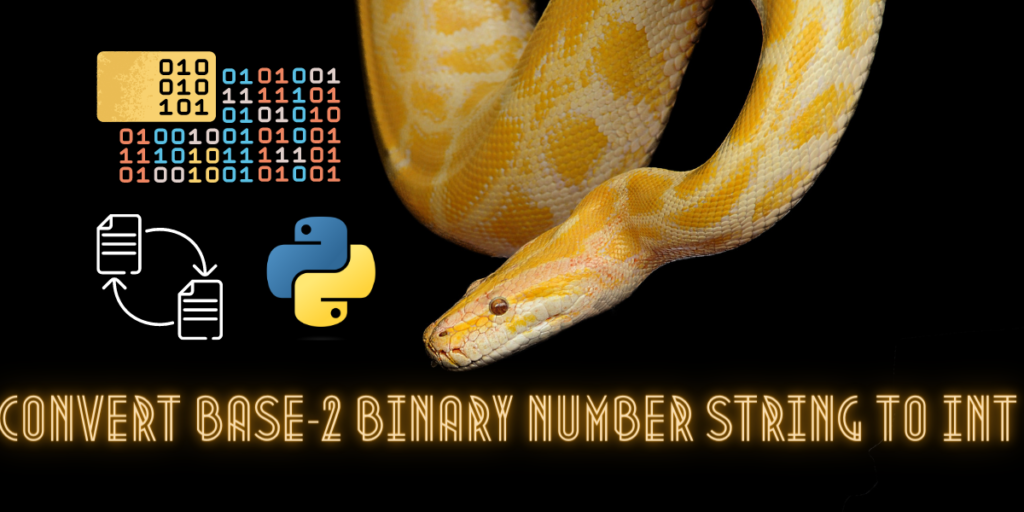# Converting Base-2 Binary Number Strings to Integers in PythonEver faced the challenge of converting a binary number string into an integer for smooth processing in your Python code? Python offers seamless solutions for transforming data types without a hitch, making it an ideal choice for developers worldwide.

In this article, we’ll delve into two popular methods to tackle binary string conversion: Python’s built-in int() function and the powerful bitstring library, part of the bit array package. Join us as we break down each approach, guiding you through the process of efficient typecasting in Python.

## What is a base-2 number string?

A binary number is expressed in the base-2 number system using only “0”s and “1”s. The zeros and ones are called the index of a binary number. They are also called “bits”.

A computer system only uses the binary system for computations. It is the way in which machine code is written. Circuit diagrams can be used to represent the working of binary systems.

Boolean algebra including “AND”, “OR” and “NOT” gates can be used to represent addition, subtraction, multiplications in the binary system.

We can easily convert a number that is an integer from the decimal system, that is the base-10 system to the binary system by dividing it with 2 and arranging the remainders in a bottom-to-top order.

A binary number can also be converted easily into a decimal number in mathematics.

A string in python is something that is written between two quotation marks, for example, “this is a string”.

Hence, a base-2 number string is a binary number written between two quotation marks . For example-‘1010001’ is a base-2 number string.

Suggested: Python bytes().

## Advantages of Explicit Typecasting in Python

In python, programmers can clearly define the conversion of one data type into another in their code. This is known as explicit typecasting in python.

Explicit typecasting is extremely useful. Unlike other programming languages, python has built-in functions that can perform explicit typecasting without having to write huge blocks of code for transforming one data type into another.

Explicit typecasting has many advantages. Some of them are:

• It helps in converting lower data types into higher ones for ease of operations.
• It prevents the loss of data when converting from one data type to another.
• Functions like int() and str() are extremely helpful and hassle free to use.

Also check out: Three ways of cubing a number in python.

## Method 1: Converting Base-2 Number String to Integer Using int() Function

We can use the int() built-in function to convert a string literal into an integer in python. Let’s look at how we can implement this :

```#converting a base-2 number string into an integer.
#taking an input for a number in the form of a string
inin=input("Enter a binary number= ")
#displaying the input
print("The given input is=",inin)
#converting using int()
outout=int(inin,2)
#displaying the output
print("the number in the decimal system or as an integer is=",outout)
```

The output would be:

```Enter a binary number= 10011
The given input is= 10011
the number in the decimal system or as an integer is= 19
```

## Method 2: Converting Base-2 Number String to Integer Using BitString Library

The bitstring module helps in natural and easy creation of binary data. Binary data analysis and manipulation using bitstring comes in very handy along with the BitArray class.

Before we can use this module we have to install in it our system, run the following code in your command prompt:

```pip install bitstring
```

Let’s see how we can implement this:

```#converting a base-2 number string into an integer.
#importing required modules
from bitstring import BitArray
#taking an input for a number in the form of a string
inin=input("Enter a binary number= ")
#displaying the input
print("The given input is=",inin)
#converting using bitArray
outout=BitArray(bin=inin).int
#displaying the output
print("the number in the decimal system or as an integer is=",outout)
```

The output will be:

```Enter a binary number= 0100111
The given input is= 0100111
the number in the decimal system or as an integer is= 39
```